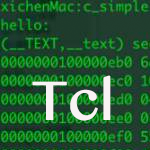# TCL过程

```proc procedureName {arguments} {
body
}```

```#!/usr/bin/tclsh

proc helloWorld {} {
puts "Hello, World!"
}
helloWorld```

```Hello, World!
```

## 过程的多个参数

```#!/usr/bin/tclsh

return [expr \$a+\$b]
}

`40`

## 过程的可变参数

```#!/usr/bin/tclsh

proc avg {numbers} {
set sum 0
foreach number \$numbers {
set sum  [expr \$sum + \$number]
}
set average [expr \$sum/[llength \$numbers]]
return \$average
}
puts [avg {70 80 50 60}]
puts [avg {70 80 50 }]```

```65
66
```

## 过程使用默认参数

```#!/usr/bin/tclsh

proc add {a {b 100} } {
return [expr \$a+\$b]
}

```40
110
```

## 递归过程

```#!/usr/bin/tclsh

proc factorial {number} {
if {\$number <= 1} {
return 1
}
return [expr \$number * [factorial [expr \$number - 1]]]

}
puts [factorial 3]
puts [factorial 5]```

```6
120```